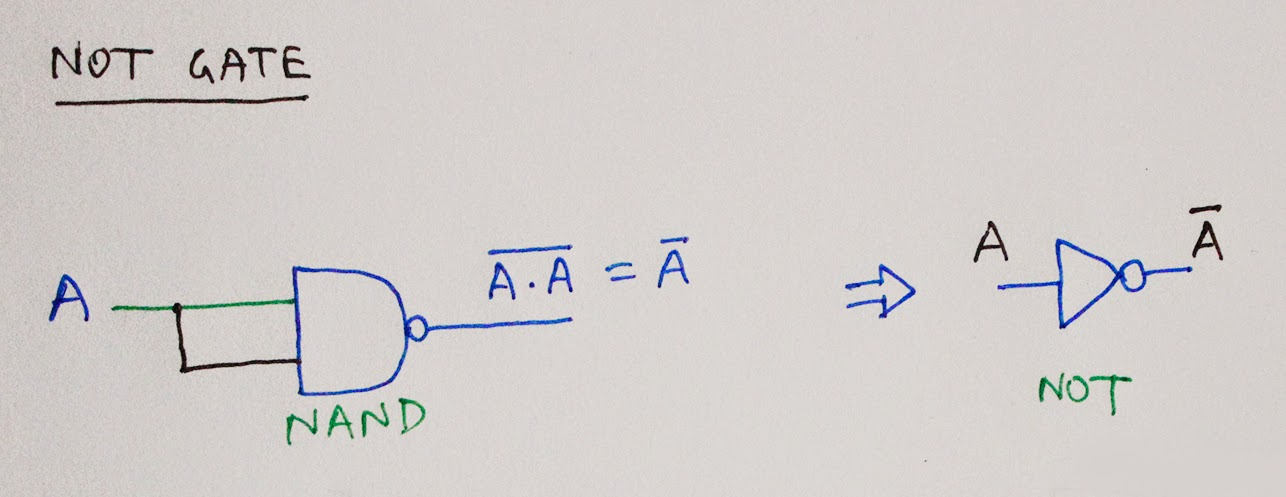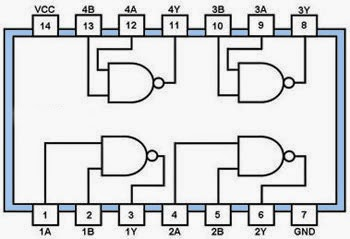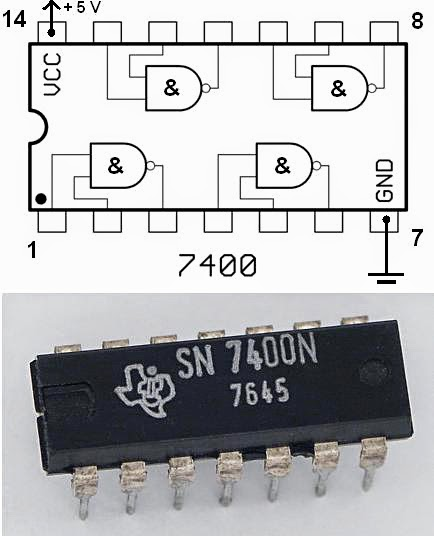Saturday, May 31, 2014

NAND Gate

Hello everyone! Welcome back again! This post is going to be about NAND gate.

So what is a NAND Gate?

In digital electronics, a NAND gate (Which can also be called Negated AND or NOT AND) is a logic gate which produces an output that is false only if all its inputs are true; thus its output is complement to that of the AND gate. You can simply think of a NAND gate like this, add a NOT gate in front of an AND Gate.

Now we had three basic logic gates which are the AND, OR and NOT, why do we need NAND?
Because we can implement any logic gates or any Boolean logic using NAND Gate.

Why NAND Gate?

NAND gate is called one the universal gates, its because we can make any Basic Gate using NAND gate(which I will show you shortly). So what is the advantage here? Clearly one of the advantages is that we can use one single logic gate to implement everything, rather than using many different types of gate, using one gate is easier. Another advantage can be reduction in size, we can reduce AND + NOT to just a NAND, lower number of gate will result in lower power dissipation and heat.

Symbol:

Its symbol is pretty much the same as that of a AND gate, only a not bubble in-front of it.Symbol of NAND Gate

Truth Table:

So lets take a look at the NAND Truth table. Like I said earlier its just the complement of the AND gate so guess you can already figure it out by now.NAND Gate Truth Table
Realization:

From the truth table using using Boolean Expression it is evident that we can treat NAND gate as an inverted input OR gate or we can just use an AND gate with a NOT in front of it.NAND Gate
So you can see that how a NAND Gate can easily be constructed using an AND gate followed by a NOT gate. From Boolean expression it can be seen that if we invert the inputs and add those inverted inputs using an OR gate we will get a NAND gate, that is also shown.

Constructing Basic Gates Using NAND Gate :

NOT GATE:NOT Gate Using NAND Gate
If we hook up both the inputs of a NAND gate give an input there we will get an output which is similar to a not gate. The Bar sign represents NOT operation.

AND GATE:AND GATE Using NAND Gate
Like I was saying earlier we can just hook up the NAND output to a NOT gate to gate an AND Gate. The output of a NAND gate is already invert of that of an AND gate, if we invert it again we will get the output of an AND gate. Simple thing if we invert something twice it stays the same. And for NOT gate we will use another NAND gate which is described above.

OR GATE:OR GATE Using NAND Gate
Now things have become a bit interesting! Like I have said earlier, from Boolean expression we can see that the output of an NAND gate is similar to that of an OR only the inputs need to be inverted. We have done exactly the same thing here. Two NAND gates with the inputs A and B acts as NOT gate, then it is fed into another NAND gate and from Boolean Expression we can see that it will act like a OR Gate.

XOR Gate:XOR Gate Using NAND Gate
XOR gate is actually exclusive OR gate which compares the two inputs. If both the inputs are same it will result in a LOW or 0 and if both the inputs are different it will result in HIGH or 1. It can be easily understood from the Truth Table. This gate is used for comparing values. We can easily construct this GATE using NAND gate.

NAND Gate Using Transistor:NAND Gate Using Transistor
So how to construct NAND gate? This gate can easily be constructed. With only two NPN transistor we can construct a NAND gate. Now let me walk you through 4 possible input combination and their output.

First of all A and B both 0. So none of the Transistors will conduct, thus we will get a certain amount of voltage on the output. So when both inputs are 0 the output will be 1.

Second, if any of the outputs are 1. If any of the outputs are 1, that transistor will conduct and the other will not conduct and as they are connected in series, no current will actually flow through them. As a result we will stil get a voltage on the output. So for any of the inputs being 1 and other being 0 we will get 1.

Finally if both the inputs are 1. If both the inputs are 1, both the transistor will conduct and thus their Emitter to collector resistance will fall to almost 0( In practical, it won't be zero). So, the output point can be treated as the Ground or 0V. So for Input 1 on both input terminal it will result in 0.

[Here 1 means presence of some amount of voltage]

NAND Gate IC

We can use NAND IC for this purpose too. They are readily available. All these ICs are of 14 pin, and can be found in both CMOS(Complementary metal–oxide–semiconductor) and TTL(Transistor Transistor Logic)

For CMOS we can use

• 4011: Quad 2-input NAND gate
• 4023: Triple 3-input NAND gate
• 4012: Dual 4-input NAND gate
• 4068: Mono 8-input NAND gateInternal Configuration of a CMOS CD4011
For TTL we can use

• 7400: Quad 2-input NAND gate
• 7410: Triple 3-input NAND gate
• 7420: Dual 4-input NAND gate
• 7430: Mono 8-input NAND gateInternal Configuration of a 7400IC
Benefit of using a CMOS is lower power consumption and better accuracy but CMOS is more prone to getting damaged.IC 7400 Which is a Quad 2 Input NAND
Anyway that's pretty much everything about NAND Gate. Hope you will find it interesting and helpful.

Take a look at my other posts here if you want

And have a nice day!